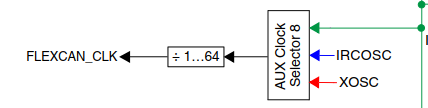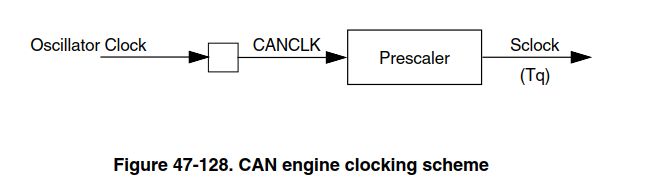## Flex CAN BIT Time and CTRL1 register values for MPC5746

cancel
Showing results for
Did you mean:
SOLVED

## Flex CAN BIT Time and CTRL1 register values for MPC5746

532 Views
Contributor V

Hi,

The example uses the value 0x01ED0006 for CAN_CTRL1 registers. A comment in the code says the values for the registers bits have been derived using /* CAN bus: 20 MHz clksrc, 500K bps with 20 tq */

Deciphering the register value, the parameters are:

PRESDIV = 1.

PSEG1 = PSEG2 =5;

RJW = 3

PROP_SEG = 6;

I checked the clock configuration in the example.

// AUX_8 clock dividers //
// FlexCAN clock devider = 5 -> 200MHz/5 = 40MHz
// Enable divider | divide by 5
MC_CGM.AC8_DC0.R = 0x80000000 | 0x40000;
MC_CGM.AC8_SC.B.SELCTL =2; //connect PLL0 on AUX_8

This gives a CAN_CLK is 40MHz. If PRESDIV =1, FSClock = (40/2) or 20MHz.

I looked at AN1798 but am not able to arrive at the same value of register parameters as used in this example.

Can someone explain how to arrive at the values used in the example? What bus length and delay parameters  are used?

What does "20 tq" mean - does it mean Nominal Bit Time (Time Quanta per bit) is 20tqs or does it mean that tq(the resolution of FSClock(Can serial clock) ) is 20ns.

Tags (1)
1 Solution
110 Views
Contributor V

Thank you so much for confirming.

Best,

Jyothsna Rajan

7 Replies
110 Views
Contributor V

I looked at the MPC5748G Reference manual and the  MPC5746 Reference manual. In MPC5746R, the field  CTRL1[CLK_SRC]  does not exist. That value is now specified in clock configuration as divider value for AuxClock8.

If the SerialClock is 20MHz and CAN_CLK is 40MHz, the value of PRESDIV should be 1.That matches the value used in code. Thanks for clarifying.

However, if I use the above values in the calculations described in MPC5746R RM Rev6, Pg1919, I cannot arrive at the register value used in the example.  Have some followup questions:

1. If PE_Clock (what documents call "CAN serial Clock") is  20MHz, and CAN_Bit_Rate is 500Kbps, the Nominal bit time comes out to 40.  But the example uses a Nominal bit time of 20 for all further calculations. Can you please explain?

2. Is the value you specified - 255us, the total_transmission_delay  or  the bus_propagation_delay ?

Per AN1798,  the value of PROP_SEG field of register CTRL1, is determined by

PROP_SEG = (total_transmission_delay) / TimeQuantum.

total_transmission_delay = (physical Interface Rx/Tx delay) + bus_propagation_delay * bus_length.

3.Is the delay value 255us? Typical delays are stated in ns.

110 ViewsNXP TechSupport

Hi,

you are right, I have referred to wrong manual. On the MPC5746R the CAN clocking is little bit different, as shown in below pictures taken from RM.Here the FLEXCAN_CLK, Oscillator Clock and CANCLK are the same signal, just different naming.

So CAN_CLK, which is used for calculation, can be sourced from divided version of PLL0, IRCOSC or XOSC.

In the example you pointed the PLL0/5 is selected so CAN_CLK is 40MHz. The bit timing selected (NBT=20tq) does not provide 500kbps, but 1Mbps.

To get 500kbps you can use this setting

CANx_CR = 0x03ED0006;  // bitrate=500kbps, CAN clk=40 MHz; Prescaler= 4, PRORSEG=7,PSEG1=6, PSEG2=6, RJW=4

255us (of course should be 255ns) is max propagation delay of single transceiver.

BR, Petr

110 Views
Contributor V

Hi Peter,

Thank you for responding.

Can you please tell me how you arrive at the values PRORSEG=7, PSEG1=6,

PSEG2=6, RJW=4?

Also, the maximum value allowed for RJW is 3 since it is only two bit

value.

Best,

Jyothsna Rajan

110 ViewsNXP Employee

Joy  Cant you can go back and edit old post and remove stuff if needed?110 ViewsNXP TechSupport

Hi,

this is calculated values, programmed are minus 1.

assume 1m bus and transceiver prop delay 255ns

1. tPROP_SEG = 2(1*5ns + 255ns) = 520 ns

2  NBT  * Prescaler = CPI_clock /baud rate = 40 MHz / 500kbps = 80

There can be 4 possibilities:

NBT = 20, Prescaler = 4

NBT = 16, Prescaler = 5

NBT = 10, Prescaler = 8

NBT =   8, Prescaler = 10

Lets choose the first one

3. PROP_SEG = ROUND_UP(520ns * 40MHz / 4) =  6

4. NBT  – 1 – PROP_SEG = 13, as the result is odd increase PROP_SEG and recalculate

20 – 1 – 7 = 12, divide the number by two and assign the result to PHASE_SEG1 and PHASE_SEG2.

PHASE_SEG1 = 6 and PHASE_SEG2 = 6

5. RJW is chosen as the smaller of 4 and PHASE_SEG1, so RJW = 4

In summary

Prescaler  = 4

Nominal Bit Time  = 20

PROP_SEG  = 7

PHASE_SEG1  = 6

PHASE_SEG2  = 6

RJW  = 4

BR, Petr

111 Views
Contributor V

Thank you so much for confirming.

Best,

Jyothsna Rajan

110 ViewsNXP TechSupport

Hi,

the example is using the XTAL as the source for the protocol clock; CTRL1[CLK_SRC] is cleared.

The EVB, on which the example was tested, is using 20MHz crystal. So the CAN bit timing is calculated based on following parameters...

PEclock=20MHz, bitrate=500kbps, bus length 1m, transceiver prop delay 255us.

20tq means Nominal Bit time, as you wrote, 20 time quantas per bit.

BR, Petr